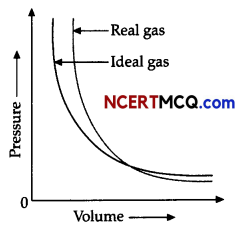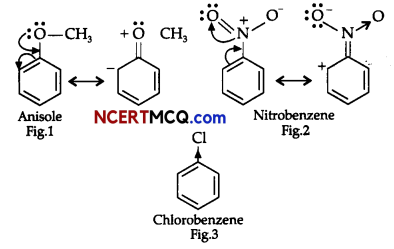Students can access the CBSE Sample Papers for Class 11 Chemistry with Solutions and marking scheme Term 2 Set 3 will help students in understanding the difficulty level of the exam.

## CBSE Sample Papers for Class 11 Chemistry Standard Term 2 Set 3 for Practice

Max. Marks: 40
Time: 2 Hours

General Instructions:

• There are 12 questions in this question paper with internal choice.
• SECTION A-Q. No. 1 to 3 are very short answer questions carrying 2 marks each.
• SECTION B-Q. No. 4 to 11 are short answer questions carrying 3 marks each.
• SECTION C- Q. No. 12 is case based question carrying 5 marks.
• All questions are compulsory.
• Use of log tables and calculators is not allowed

Section – A

Question 1.
Arrange the following in the increasing order of their property indicated (any 2):
(i) Lithium, Sodium, Rubidium, Potassium (ionization enthalpy)
(ii) MCI, MBr, MF, MI (where M = alkali metal)(covalent character)
(iii) Mg(OH)2, Sr(OH)2, Ba(OH)2, Ca(OH)2 (Increasing solubility in water)

Question 2.
At 0°C, the density of a gaseous oxide at 2 bar is the same as that of nitrogen at 5 bar. What is the molecular mass of the oxide?

Question 3.
(i) pH of our blood remains almost constant at 7.4 though we quite often eat spicy food.
(ii) Ammonia termed as a base though it does not contain OH ions.Section-B

Question 4.
Account for the following:
(i) Boiling points of alkane decreases with increase in the branching.
(ii) Nitration of benzene with nitric acid requires the use of concentrated sulphuric acid.
(iii) Cycloalkanes are isomeric with alkenes.
OR
Convert the following organic compounds:
(i) Ethene into benzene
(iv) Benzene into p-nitrobromobenzene.

Question 5.
(i) At 500 K, equilibrium constant, Kc for the following reaction is 5.
$$\frac{1}{2}$$ H2(g) + $$\frac{1}{2}$$ I2 (g) ⇌ HI(g)
What would be the equilibrium constant Kc, for the reaction 2HI (g) ⇌ H2 (g) + I2(g)?
(ii) (a) What is pH? What is its value for addic solution?
(b) What is pOH? What is its value for neutral water at 25°C?
OR
(i) What happens to the pH of buffer solution on dilution?
(ii) (a) Why is BF3 termed as an acid though it does not contain H+ ions?
(b) The ionic product of water is 0.11 x 10-14 at 273 K, 1 x 10-14 at 298 K and 7.5 x 10-14 at 373K.
Deduce from this data whether the ionisation of water to hydrogen and hydroxide ion is exothermic or endothermic?Question 6.
Account for the following:
(i) Concentrated HNO3 can be transported in aluminum container.
(ii) BF3 is reacted with ammonia.
(iii) Graphite is used as lubricant.

Question 7.
Draw the Lewis structure of O2 ion and calculate oxidation state of oxygen atom. What is the average oxidation state of oxygen in this ion?

Question 8.
Observe the Pressure vs volume graph for a real gas and an ideal gas and answer the questions given below:(i) Interpret the behaviour of real gas with respect to ideal gas at low pressure.
(ii) Interpret the behaviour of real gas with respect to ideal gas at high pressure.
(iii) Mark the pressure and volume by drawing a line at the point where real gas behaves as an ideal gas.

Question 9.
What happens when following chemical reactions occur:
(i) HBr is added to hex-1-ene in absence of peroxide.
(ii) Ozonolysis of 1,2-dimethylbenzene is carried out.
(iii) Phenol is heated with zinc dust.
OR
Sample Question Papers
(i) Give the IUPAC name for the following alkane.(ii) Complete the following chemical reactions:Question 10.
Compare the solubility and thermal stability of the following compounds of the alkali metals with those of the alkaline earth metals:
(i) Nitrates
(ii) Carbonates.Question 11.
(i) Presence of nitro group makes the benzene ring less reactive in comparison to the unsubstituted benzene ring. Why?
(ii) Why is Wurtz reaction not preferred for the preparation of alkanes containing odd number of carbon atoms?
(iii) Why is rotation around carbon-carbon single bond of ethane is not completely free?
OR
On the basis of the figure given below, answer the following questions:(i) Which effect is shown by the substituted group in Fig. 1?
(ii) Which effect is shown by the substituted group in Fig. 2?
(iii) Which effect is shown by the substituted group in Fig. 3?

Section – C

Question 12.
Decomposition of ammonia to hydrogen (and nitrogen) requires an energy input, and since the decomposition reaction is very slow even at relatively high temperatures, a catalyst is also needed. Since the reaction is an equilibrium reaction, it is not possible to completely decompose ammonia into hydrogen. Maximizing the efficiency of a complete fuel cell system utilizing metal amines for hydrogen storage,therefore, requires both efficient heat integration to minimize losses and a properly designed catalyst system to achieve a sufficiently fast hydrogen production rate.

For fuel cell systems that are not tolerant to ammonia, it is also necessary to include an ammonia scavenger. These units are already well known in the chemical industry. Historically, ammonia decomposition has received most of its attention as a model reaction studied to gain fundamental insight into the technically important ammonia synthesis reaction (Hansen, 1995).

However, a few industrial ammonia decomposition plants are currently in operation to produce deuterium-enriched ammonia by coupling consecutive synthesis- decomposition cycles combined with distillation. These plants operate at moderate pressures but at quite high temperatures (600 °C).
(i) At 25°C, the equilibrium constant K1 and K2 are for the reactions:Show the relation between two equilibrium constants.(ii) On increasing the pressure, in which direction will the gas phase reaction proceed to re-establish equilibrium, is predicted by applying the Le Chatelier’s principle. Consider the reaction.
N2(g) + 3H2(g) ⇌2NH3(g)

(iii) In which of the following reactions, the equilibrium remains unaffected on addition of small amount of argon at constant volume?
(A) H2(g) + I2(g)⇌2HI(g)
(B) PCl5(g)⇌PCl3(g) + Cl2(g)
(C) N2(g)+3H2(g) ⇌ 2NH3(g)

(iv) For the reaction, N2(g) + 3H2(g) ⇌ 2NH3(g), the partial pressures of N2 and H2 are 0.80 atm and 0.40 atm respectively at chemical equilibrium. The total pressure of the system is 2.80 atm. Calculate the value of Kp.
OR
The following concentrations were obtained for the formation of NH3 from N2 and H2 at equilibrium at 500K, [N2] = 1.5 X 10-2M,
[H2] = 3.0 x 10-2 M and [NH3] = 1.2 x 10-2M.
Calculate equilibrium constant.